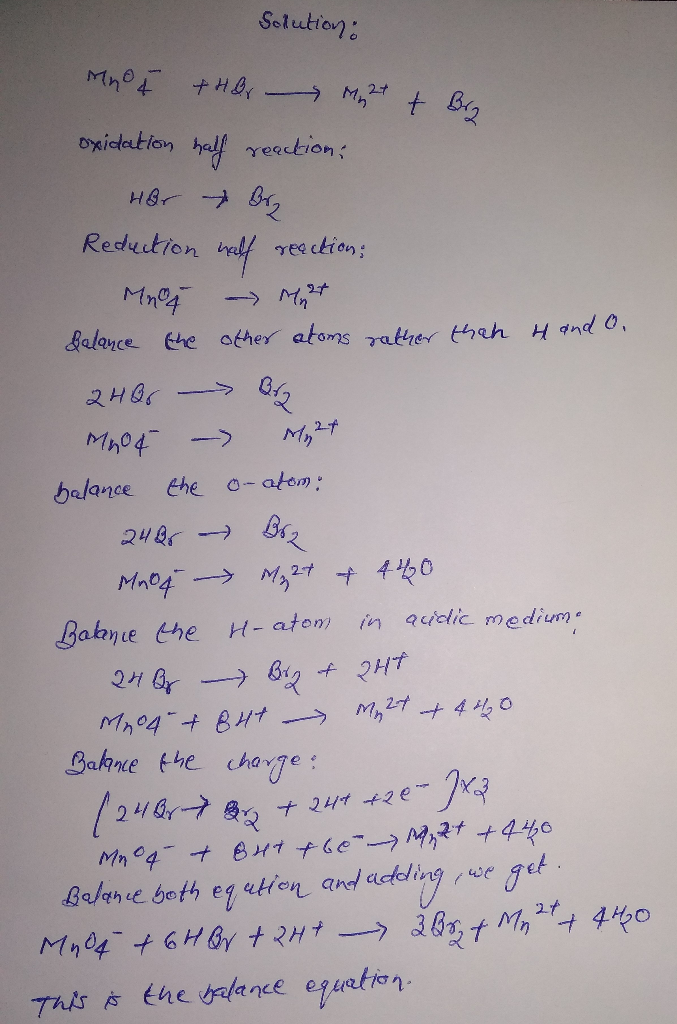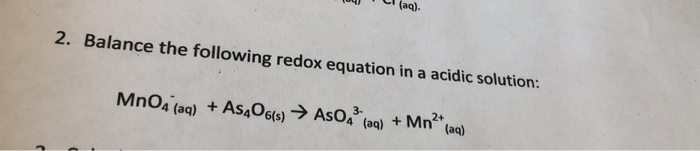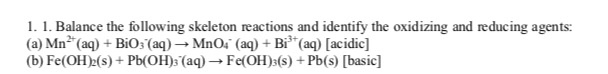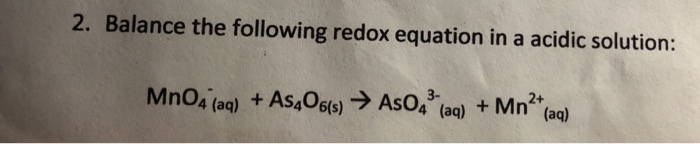Question

# BALANCE: MnO4-1 + HBr > Mn+2 + Br2 in acidic media

BALANCE:

MnO4-1 + HBr > Mn+2 + Br2 in acidic media#### Earn Coins

Coins can be redeemed for fabulous gifts.

Similar Homework Help Questions
• ### Balance Redox Equations (Basic Solution) with steps. 1. Mn^2+ (aq) + Br2(l) = MnO2 (s) + Br^- (aq) 2. NO2^- (aq) + MnO4^...

Balance Redox Equations (Basic Solution) with steps. 1. Mn^2+ (aq) + Br2(l) = MnO2 (s) + Br^- (aq) 2. NO2^- (aq) + MnO4^- (aq) = NO3^- (aq) + MnO2 (s) 3. N2H4 (g) + ClO3^- (aq) = NO(g) + Cl^- (aq)

• ### Balance the following redox reactions: Fe^2+ + MnO4^- + H^+ → Fe^3+ + Mn^2+ + H2O...

Balance the following redox reactions: Fe^2+ + MnO4^- + H^+ → Fe^3+ + Mn^2+ + H2O Mn^2+ + MnO4^- + …→ MnO2 + … IO3^- + I^- + H^+ → I2 + H2O

• ### 1. Balance the following equation in an acidic environment HCOOH + MnO4-  CO2 + Mn2+

1. Balance the following equation in an acidic environment HCOOH + MnO4-  CO2 + Mn2+

• ### Permanganate, MnO4–, can act as an oxidizing agent in both acidic and basic aqueous solutions. Balance each of the following reactions by the half–reaction method, showing all steps in the balancing process.

Permanganate, MnO4–, can act as an oxidizing agent in both acidic and basic aqueous solutions.  Balance each of the following reactions by the half–reaction method, showing all steps in the balancing process.                          i.            MnO4–   +   Al   ––>   MnO2   +   Al(OH)4–                                in aqueous base                          ii.           MnO4–   +   CH3COOH   ––>   Mn+2   + CO2                            in aqueous acid

• ### 104 ' (aq). 2. Balance the following redox equation in a acidic solution: MnO4 (aq) +...104 ' (aq). 2. Balance the following redox equation in a acidic solution: MnO4 (aq) + As406(s) → Asos (aq) + Mn2+ (aq)

• ### 1. 1. Balance the following skeleton reactions and identify the oxidizing and reducing agents: (a) Mn+...1. 1. Balance the following skeleton reactions and identify the oxidizing and reducing agents: (a) Mn+ (aq) + BiO3 (aq) →MnO4 (aq) + Bit (aq) (acidic) (b) Fe(OH)2(s) + Pb(OH)3 (aq) Fe(OH)3(s) + Pb(s) (basic)

• ### Balance the equation for the following redox reaction in acidic solution MnO4- + VO2+ ----> MnO2...

Balance the equation for the following redox reaction in acidic solution MnO4- + VO2+ ----> MnO2 + VO2+

• ### 2. Balance the following redox equation in a acidic solution: MnO4 (aq) + As«O6(s) → AsO4 (aq) + Mn2+(aq)2. Balance the following redox equation in a acidic solution: MnO4 (aq) + As«O6(s) → AsO4 (aq) + Mn2+(aq)

• ### help me balance the redox equations (acidic solutions) 1) BrO3- + Br- —> Br2 2) IO3-...

help me balance the redox equations (acidic solutions) 1) BrO3- + Br- —> Br2 2) IO3- + I- —> I2 3) C2H4 + MnO-4 —> Mn2+ + CO2

• ### 1. Balance the following reaction in acidic solution. I-(aq) + MnO4-(aq)  Mn2+(aq) + I2(s) 2....

1. Balance the following reaction in acidic solution. I-(aq) + MnO4-(aq)  Mn2+(aq) + I2(s) 2. Write a balanced net ionic equation for the following reaction in basic solution using both methods for balancing a redox reaction:      Zn(s)  +  NO3-(aq)  à  NH3 (aq)  +  Zn(OH)42- (aq) 3. Write a balanced net ionic equation for the following reaction in basic solution using both methods for balancing a redox reaction:         MnO4-(aq)  + C2H5OH(aq) à Mn2+(aq) + HC2H3O2 (aq)